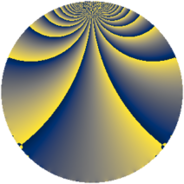# Properties

 Label 3024.1.egLevel $3024$ Weight $1$ Character orbit 3024.eg Rep. character $\chi_{3024}(53,\cdot)$ Character field $\Q(\zeta_{12})$ Dimension $16$ Newform subspaces $2$ Sturm bound $576$ Trace bound $10$

# Related objects

## Defining parameters

 Level: $$N$$ $$=$$ $$3024 = 2^{4} \cdot 3^{3} \cdot 7$$ Weight: $$k$$ $$=$$ $$1$$ Character orbit: $$[\chi]$$ $$=$$ 3024.eg (of order $$12$$ and degree $$4$$) Character conductor: $$\operatorname{cond}(\chi)$$ $$=$$ $$336$$ Character field: $$\Q(\zeta_{12})$$ Newform subspaces: $$2$$ Sturm bound: $$576$$ Trace bound: $$10$$

## Dimensions

The following table gives the dimensions of various subspaces of $$M_{1}(3024, [\chi])$$.

Total New Old
Modular forms 80 16 64
Cusp forms 32 16 16
Eisenstein series 48 0 48

The following table gives the dimensions of subspaces with specified projective image type.

$$D_n$$ $$A_4$$ $$S_4$$ $$A_5$$
Dimension 0 0 16 0

## Trace form

 $$16q + O(q^{10})$$ $$16q - 4q^{10} - 8q^{13} + 8q^{16} + 12q^{28} + 12q^{31} - 4q^{37} + 8q^{43} - 8q^{46} - 4q^{49} - 4q^{52} + 4q^{58} - 4q^{61} - 4q^{67} - 16q^{76} - 8q^{79} - 4q^{88} + 4q^{91} + 4q^{94} + 8q^{97} + O(q^{100})$$

## Decomposition of $$S_{1}^{\mathrm{new}}(3024, [\chi])$$ into newform subspaces

Label Dim. $$A$$ Field Image CM RM Traces $q$-expansion
$$a_2$$ $$a_3$$ $$a_5$$ $$a_7$$
3024.1.eg.a $$8$$ $$1.509$$ $$\Q(\zeta_{24})$$ $$S_{4}$$ None None $$0$$ $$0$$ $$0$$ $$0$$ $$q-\zeta_{24}q^{2}+\zeta_{24}^{2}q^{4}-\zeta_{24}^{7}q^{5}-\zeta_{24}^{6}q^{7}+\cdots$$
3024.1.eg.b $$8$$ $$1.509$$ $$\Q(\zeta_{24})$$ $$S_{4}$$ None None $$0$$ $$0$$ $$0$$ $$0$$ $$q-\zeta_{24}^{7}q^{2}-\zeta_{24}^{2}q^{4}+\zeta_{24}^{10}q^{7}+\cdots$$

## Decomposition of $$S_{1}^{\mathrm{old}}(3024, [\chi])$$ into lower level spaces

$$S_{1}^{\mathrm{old}}(3024, [\chi]) \cong$$ $$S_{1}^{\mathrm{new}}(1008, [\chi])$$$$^{\oplus 2}$$